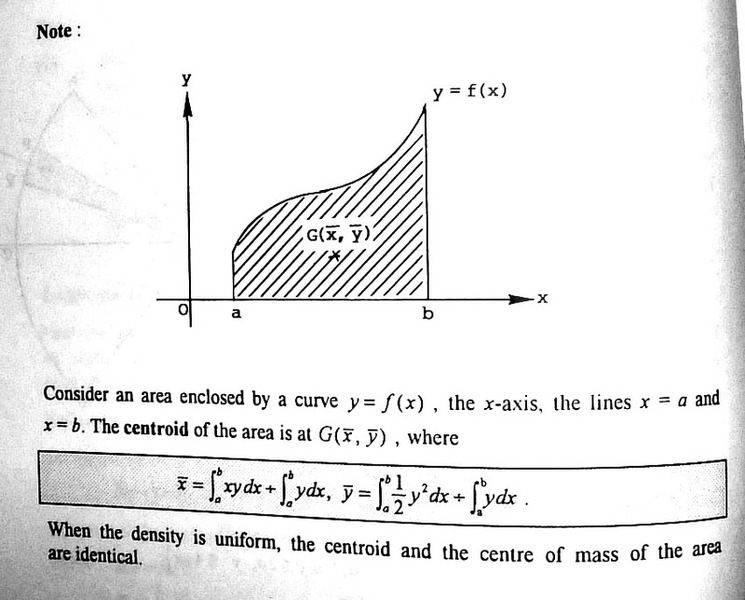# Statics and Friction 02

## Homework StatementNil

## The Attempt at a Solution

Why does the bar y have a factor of 0.5 for the forumla of area centroid?

Thank you very much!

Am I right that the + is actually an devided by?

$$\bar{y}=\frac{1}{A} \int y\ dx dy = \frac{1}{A} \int 0.5\ y^2\ dx$$

Is that ok?

cepheid
Staff Emeritus
$$\div$$​